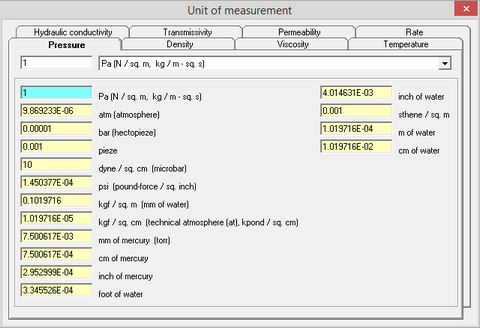﻿ Help > Unit converter (Part 1) > Pressure

# Pressure

Navigation:  Help > Unit converter (Part 1) >

# Pressure

Pressure (P) is defined as a force applied over a unit area, perpendicular to its surface.Tab "Pressure" of the dialog window "Unit converter (part 1)"

Explanatory table (Source: Wikipedia)

 Pascal (Pa) Newton per sq.m (N/m2) The pascal is the SI derived unit of pressure. The unit, named after Blaise Pascal, is defined as one newton per square metre. 1 Pa = 1 N/m2 ≈ 9.87 x 10-6 atm. To other SI units, Pa is related as: 1 Pa = 1 kg.m-1.s-2 1 Н = 1 kg.m.s-2 Standard (physical) atmosphere (atm) The standard atmosphere is a unit of pressure defined as 101325 Pa (1.01325 bar). One atm corresponds to the standard (or reference) atmospheric pressure equal to 760 mm of mercury column at 0 °C and standard gravity acceleration (g = 9.80665 m/s2).  It is approximately equal to the atmospheric pressure at sea level Bar The bar is a metric unit of pressure, but not part of the International System of Units (SI). It is defined as exactly equal to 100,000 Pa (100 kPa), which is the on earth at an altitude of about 111 meters and a temperature of 15 °C or slightly less than the current average pressure at sea level (approximately 1.013 bar) Pieze The pieze is the unit of (MTS system), used in the former1933–1955. It is defined as one 1 Pieze = 103 Pa Dyne per square centimeter (dyn/cm2) Microbar Pressure unit in CGS system. 1 dyn/cm2 = 0.1 Pa. Psi Pound per square inch The pound per square inch or, more accurately, pound-force per square inch is a unit of pressure or of stress. It is the pressure resulting from a force of one pound-force applied to an area of one square inch. In SI units, 1 psi is approximately equal to 6895 N/m2. mm of water column (mm H2O) Millimeters, water gauge, also known as a millimetre of water or millimetres water column and abbreviated to mmwg, mmH2O or mmwc, respectively, is defined as the pressure exerted by a column of water of 1 mm in height at 4 °C (temperature of maximum density) at the standard acceleration of gravity, so that 1 mmH2O (4°C) = 999.9720 kg/m3 × 9.80665 m/s2 × 1 mm = 9.8063754138 Pa ≈ 9.80638 Pa, but conventionally a nominal maximum water density of 1000 kg/m³ is used, giving 9.80665 Pa. Technical atmosphere (at) The technical atmosphere (symbol: at) is a non-SI unit of pressure equal to one kilogram-force per square centimetre. 1 at = 98.0665 kPa ≈ 0.96784 standard atmospheres Millimeter of mercury column (mm Hg) A millimetre of mercury is a manometric unit of pressure, formerly defined as the extra pressure generated by a column of mercury one millimetre high, and currently defined as exactly 133.322387415 pascals. It is denoted mmHg or mm Hg. Although not an SI unit, the millimetre of mercury is still routinely used in medicine, meteorology, aviation, and many other scientific fields. One millimetre of mercury is approximately 1 Torr, which is 1/760 of standard atmospheric pressure (101325/760≈ 133.322368421053 Pa). Although the two units are not equal, the relative difference (less than 0.000015%) is negligible for most practical uses. Centimeter of mercury column (cm Hg) 1 cm Hg = 10 mm Hg ≈ 1333.224 Pa Inch of mercury column (in Hg) 1 in Hg = 25.4 mm Hg ≈ 3386.379 Pa ft of water column (ft H2O) 1 ft H2O = 304.8 mm H2O = 2989.06692 Pa inch of water column (in H2O) 1 in H2O =  25.4 mm H2O = 249.08891 Pa Sten per square meter Sten - is a unit of force that was used in the MTS system in the Soviet Union between 1933 and 1955. 1 sten equals to the force that is applied to the mass of 1 ton and results in its acceleration of 1 m/s2. 1 sten = t·m/s2 = 103 N. 1 sten/m2 = 1000 Pa m of water column (m H2O) 1 m H2O = 0.1 аt